# CBSE Class 10 Science Electricity Assignment Set B

Read and download free pdf of CBSE Class 10 Science Electricity Assignment Set B. Get printable school Assignments for Class 10 Science. Standard 10 students should practise questions and answers given here for Chapter 12 Electricity Science in Grade 10 which will help them to strengthen their understanding of all important topics. Students should also download free pdf of Printable Worksheets for Class 10 Science prepared as per the latest books and syllabus issued by NCERT, CBSE, KVS and do problems daily to score better marks in tests and examinations

## Assignment for Class 10 Science Chapter 12 Electricity

Class 10 Science students should refer to the following printable assignment in Pdf for Chapter 12 Electricity in standard 10. This test paper with questions and answers for Grade 10 Science will be very useful for exams and help you to score good marks

### Chapter 12 Electricity Class 10 Science Assignment

Question. What is the current through a 5.0 ohm resistor if the voltage across it is 10 V
(a) zero
(b) 0.5 A
(c) 2.0 A
(d) 5.0 A

Question. 100 J of heat is produced each second in a 4 W resistance. The potential difference across the resistor is
(a) 20 V
(b) 10 V
(c) 5 V
(d) 15 V

Question. A cylindrical conductor of length l and uniform area of cross-section A has resistance R. Another conductor of length 2l and resistance R of the same material has area of cross section
(a) A/2
(b) 3A/2
(c) 2A
(d) 3A

Question : An electric bulb is rated 220 V and 100 W. When it is operated on 110 V, the power consumed will be:
a. 100 W
b. 75 W
c. 50 W
d. 25 W

Question : A student uses as battery of adjustable voltage 0-6 V. she has to perform an experiment to determine the equivalent resistance of two resistors when connected in parallel using two resistors of value 3 ohm and 6 ohm. The best choice of combination of voltmeter and ammeter to be used in the experiment is
a. Ammeter of range 0-5 A and voltmeter of range 0-2 V
b. Ammeter of range 0-2 A and voltmeter of range 0-10 V
c. Ammeter of range 0-5 A and voltmeter of range 0-5 V
d. Ammeter of range 0-5 A and voltmeter of range 0-10 V
Explanation: Voltmeter has to read beyond 6 V and with 0-2A range, ammeter will have a better least count but net current drawn for 6V is 3A.

Question : Students A and B connected the two resistors R1 and R2 given to them in the manners shown below :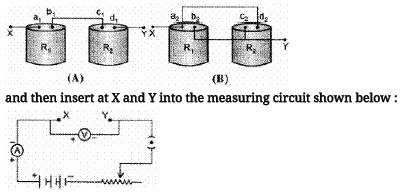We can say that
a. both the students will determine the equivalent resistance of the series combination of R1 and R2.
b. Student A will determine the equivalent resistance of the series combination while student B will determine the equivalent resistance of the parallel combination of  R1 and R2.
c. both the student will determine the equivalent resistance of the parallel combination of  R1 and R2.
d. student A will determine the equivalent resistance of the parallel combination while student B will determine the equivalent resistance of the series combination of  R1 and R2.

Explanation: c1, b1 is the common node in A so series b2, c2 pair and a2, d2 pair of points are at same potential. So parallel in B.

### Assertion and Reason

DIRECTION : In the following questions, a statement of assertion (A) is followed by a statement of reason (R). Mark the correct choice as:
(a) Both assertion (A) and reason (R) are true and reason (R) is the correct explanation of assertion (A).
(b) Both assertion (A) and reason (R) are true but reason (R) is not the correct explanation of assertion (A).
(c) Assertion (A) is true but reason (R) is false.
(d) Assertion (A) is false but reason (R) is true.
(e) Both Assertion and Reason are false.

Question. Assertion : The resistivity of conductor increases with the increasing of temperature.
Reason : The resistivity is the reciprocal of the conductivity.

Question. Assertion : A voltmeter and ammeter can be used together to measure resistance but not power.
Reason : Power is proportional to voltage and current.

Question. Assertion : The 200 W bulbs glow with more brightness than 100 W bulbs.
Reason : A 100 W bulb has more resistance than 200 W bulb.

Question. Assertion : When current through a bulb decreases by 0.5% the glow of bulb decreases by 1%.
Reason : Glow (Power) which is directly proportional to square of current.

Question. Assertion : Two resistance having value R each. Their equivalent resistance is = R/2.
Reason : Given Resistance is connected in parallel.

Question : Write S.I. unit of resistivity.

Question : Define resistance. Give its S.I. unit.
Answer :  Resistance is the property of a conductor to ppose the flow of charges through it. SI unit of resistance is Ohm (Ω).

Question. State Joule’s law of heating.
Answer: Joule’s law of heating states that the amount of heat produced in a conductor is directly proportional to:
(a) Square of current (I2)
(b) Resistance of wire (R)
(b) Time (t), for which current is passed.

Question. Define electric power.
Answer: The electrical work done per unit time is called electric power.

Question. What does an electric circuit mean?
Answer: A continuous conducting path consisting of wires and other electrical components (like resistance or electric bulb, switch etc.) between the two terminals of a cell or battery, along which an electric current flows, is called an electric circuit.

Question. Distinguish between an open and a closed circuit.
Answer: An electric circuit is said to be an open circuit when the switch is in ‘off’ mode (or key is unplugged) and no current flows in the circuit.
The circuit is said to be a closed circuit when the switch is in ‘on’ mode (or key is plugged) and a current flows in the circuit.

Question. How many 220 Ω resistors (in parallel) are required to carry 5 A on a 220 V line ?

Question. Several electric bulbs designed to be used on a 220 V electric supply line, are rated 10W. How many lamps can be connected in parallel with each other across the two wires of 220 V line if the maximum allowable current is 5 A ?

Question. Which uses more energy :
(a) a 250 W T.V. set in 1 hour
(b) a 1200 W toaster in 10 minutes ?
Answer: (a) 9 × 105 J, (b) 7.2 × 105 J (T.V. set uses more energy)

Question. Study the circuit diagram given in figure carefully and calculate :
(a) Current in main circuit.
(b) Current in each of the resistors in the parallel circuit.
Answer: (a) 1.80 A, (b) 1.2 A and 0.6 A, 1.2 A and 0.6 A.

Question. A wire of resistance 1.5 Ω is stretched to double its length. What will be its new resistance ?

Question. A wire of resistance 36 Ω and length 60 cm is tripled on itself. What is the new resistance ?

Question. How many electrons are flowing per second past a point in a circuit in which there is a current of 1 A ?

Question. The graph between V and I for a conductor is a straight line passing through the origin.
(a) Name the law illustrated by such a graph ?
(b) What should remain constant in a statement of this law ?
Answer: (a) Ohm's law, (b) Temperature.

Question : The potential difference across the wire having fixed resistance is tripled. By how much does the electric power increase?
Answer :  The electric power will increase by nine times when the potential difference across the wire having fixed resistance is tripled. According to Ohm’s law, potential difference V is proportional to current, I. Therefore, when V is made 3 times, I will increase 3 times. As Power P = VI, therefore, Power will increase by 9 times.

Question : find the minimum rating of fuse that can be safely used on a line on which two 1.1 KW electric geysers are to run simultaneously.
The supply voltage is 220 V.
Answer :  Power P = VI. As the two geysers have power rating 1.1 kW or 1100 W and are connected in parallel, each geyser draws a current I = P/V = 1100/220 A = 5 A.

Question : Should the resistance of an ammeter be low or high? Give reason.
Answer :  An ideal ammeter is one which has zero resistance. But that is not possible. Therefore, the resistance of an ammeter should be as close to zero as possible. If it is non-zero and
substantial, it will affect the current flowing through the circuit. This is because an ammeter is connected in series in the circuit for the measurement of electric current.

Question : The electric power consumed by a device may be calculated by either of the two expressions P = I2R or P = V2/R. The frist expression indicates that it is directly proportional to R whereas the second expression indicates inverse proportionality. How can the seemingly different dependence of P on R in these expressions be explained?
Answer :  The expression P = I2R is used for calculating electric power when only current I and resistance R are known, whereas P = V2/R is used for calculating power when voltage V and
resistance R are known.

Question : Define the unit of electric current.
Answer :  The SI unit of electric current is ampere (A). When 1 coulomb of electric charge flows through any cross-section of a conductor in 1 second, the electric current flowing through it is said to be 1 ampere.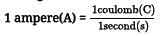Question : How many joule are there in 1 kWh?
1kWh = 1,000 Ω × 1h = 1,000 J/S × 3600S
1kWh = 3,600,000 J = 3.6 ×106 J

Question. The given figure represents V-I graph for a series combination and for a parallel combination of two resistors. Which of the two, A or B, represents the series combination. Give a reason for your answer.Answer: Since, the straight line A is steeper than B, so the straight line A represents a greater resistance. The equivalent resistance in a series combination is greater than in parallel combination. Hence, A represents series combination.

Question. You are given three resistors of resistance 2 W, 4 W and 6 W. Show by a diagram, how you can get a 3 W resistance with the help of these resistors.
Answer: Connect 2 W and 4 W in series then this combination is connected with the parallel 6 W resistance. By this arrangement we can get resistance 3 W as equivalent resistance.

Question. Derive the relation 1/R = 1/R1 + 1/R2 + 1/R3 when resistors are joined in parallel.
Answer: In parallel combination of three resistance R1, R2 and R3, the current in each of the resistance is different. If I is the current drawn from the cell then it is divided into branches as I1, I2 and I3. Thus,
I = I1 + I2 + I3
The potential difference across each of these resistances is the same.
Thus, from Ohm’s law
I1 = V /R1 , I2 = V/R2 , I3 = V/R3
If R is the equivalent resistance then,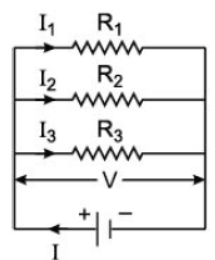V/R = V/R1 + V/R2 + V/R3
and 1/R = 1/R1 + 1/R2 + 1/R3 .

Question. How many 10 W resistors are required to get a 25 W resistor?
Answer: Four 10 W resistors are required and they are connected as shown.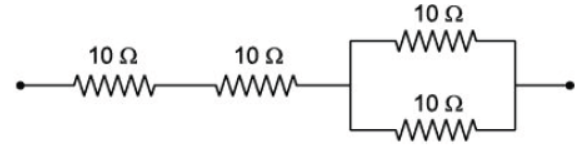Question. You are given fifty 5 W resistors. What is (a) smallest and (b) largest resistance can be obtained by using these?
Answer: (a) To get the smallest resistance, all the 5 W resistors must be connected in parallel.
Smallest resistance = 5/50 = 0.1 W
(b) To get the largest resistance, the 5 W resistors must be
connected in series.
Largest resistance = 5 × 50 = 250 W.

Question. Why are fairy decorative lights always connected in parallel?
Answer: When the fairy lights are connected in series the resistance offered will be greater and brightness of the bulbs will be affected. But in parallel connection all the bulbs will glow with same intensity and if any bulb gets fused the other bulbs will continue to glow.

Question. Resistance of a conductor of length 80 cm is 4.0 W. Calculate the resistance of a similar conductor of length 400 cm.
Answer: Here, l1 = 80 cm, R1 = 4.0 W, l2 = 400 cm, R2 = ?
∴ R1/R2 = 11/12
or R2 = R1. 1/2 , 1/1
= 4.0 × 400/80 = 20.0 W.

Question. Out of 60 W and 40 W lamps, which one has a higher electrical resistance when in use.
Answer: Power (P) = V2/R .
From the above formula, P is inversely proportional to R (resistance) as voltage remaining the same. Hence, 40 We lamp has high resistance.

Question. Write the formula for current ‘I’ flowing through a conductor if ‘n’ electrons flow through the cross-section of a conductor in time ‘t’.
Answer: If ‘n’ electrons pass through the cross-section of a conductor in time ‘t’, the total charge ‘Q’ passing through the conductor is :
Q = ne (e is the charge on an electron = 1.6 ×10–19 C) The current ‘I’ in the conductor is :
l = Q/t = ne/t

Question. What is commercial unit of electrical energy ? Convert it into joules.
Answer: The commercial unit of electrical energy is kWh.
1 kWh = 1000 W × 1 hour
= 1000 j/s x 60 x 60s
= 3.6 × 106 J

Question : Heat is generated continuously in an electric heater, but the temperature of its element becomes constant after some time. Why?
Answer :   When the temperature of the heater becomes greater than the temperature of the surroundings, some of the heat is lost to the surroundings in the form of thermal radiations and through convection. After some time,the rate at which heat is being produced becomes equal to the rate of which heat is lost. Hence, the temperature of the element becomes constant. This happens because an equilibrium or steady state is reached.

Question : Define 1 volt. Express it in terms of SI unit of work and charge calculate the amount of energy consumed in carrying a charge of 1 coulomb through a battery of 3 V.
Answer :  When 1 joule of work is done in carrying 1 coulomb of charge, from infinity to a point in the electric field, then potential at that point is called 1 volt. Potential difference between two points is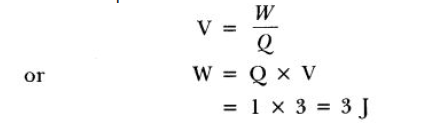Question :  A heater coil is rated 100 W, 200 V. It is cut into two identical parts. Both parts are connected together in parallel to the same source of 200 V. Calculate the energy liberated per second in the new combination.
Answer : Resistance of heater coil,As the coil is cut into two identical parts, resistance of each part = 400/2  = 200 Ω
When connected in parallel, the net resistance is given byEnergy liberated per second in the new combination is 400J

Question : Why is parallel arrangement used in domestic wiring?
Answer :  Parallel arrangement is used in domestic wiring due to the following reasons:
• Each device will have the same voltage which is equal to the voltage of the supply.
• If two or more devices are used at the same time, then each appliance will be able to draw the required current.
• If one of the devices fails, then the other keeps working.

Question :  a. Define electrical energy with the S.I. unit?
b. A household uses the following electric appliance:
(i) Refrigerator of rating 400Wfor ten hours each day.
(ii) Two electric fans of rating 80W each for twelve hours each day.
(iii) Six electric tubes of rating 18W each for six hours each day.
Calculate the electricity bill of the household for the month of June if the cost per unit of electric energy is Rs.3.00
a. The work done by a source of electricity to maintain current in a circuit is known as electrical energy. Its S.I. unit is joule.

b. i. Electricity consumed by refrigerator in one day

= power x time

= 400W x 10h

= 4000Wh

= 4kWh

ii. Electricity consumed by 2 electric fans in 1 day

= power x time

= 2 x 80W x 12h

= 1920Wh

= 1.92kWh

iii. Electricity consumed by 6 electric tubes in 1 day

= 6 x 18W x 6h

= 648wh = 0.648 kWh

Total energy consumed in one day

= 4+1.92+0.648 = 6.548 kWh

Total energy consumed in one month

= 6.568 x 30

= 197.04 kWh

Cost of 1 unit (kWh) = Rs. 3.00

Cost of 197.04 kWh = 197.04 3

The required electricity bill = Rs. 591.12

Question. How can three resistors of resistances 2 W, 3 W and 6 W be connected to give a total resistance of (a) 4 W and (b) 1 W?
Answer: (a) The three resistors of resistances 2 W, 3 W and 6 W have to be combined as shown in the figure to obtain 4 W resistance.
Equivalent resistance of 3 W and 6 W connected in parallel is, 1/Rp = 1/3 + 1/6 = 2 + 1/6 = 3/6
or RP = 6/3 = 2 W 126
Now, RP and 2 W are joined in series and the equivalent resistance is,
R = RP + 2
= (2 + 2) W
= 4 W
(b) In order to obtain 1 W resistance, the resistors 2 W, 3 W and 6 W have to be combined as shown in figure.
2 W, 3 W and 6 W resistances are connected in parallel, 126
∴ 1/R = 1/2 + 1/3 + 1/6
= 3 + 2 + 1/6
= 6/6
or R = 1 W

Question. How many 176 W resistors in parallel are required to carry 5 A on a 220 V line?
Answer: Here, Potential difference, V = 220 V, Current, I = 5 A
∴ Resistance, R = V/I
= 220/5 = 44 W
Let the number of 176 W resistors to be connected in parallel to give an equivalent resistance of 44 W be x.
Equivalent resistance of ‘x’ 176 W resistance connected in parallel is 176/x W
But, 176/x , = 44
∴ x = 176/44 = 4
Thus, 4 resistors of 176 W each should be connected in parallel.

Question. Draw a schematic diagram of a circuit consisting of a battery of three cells of 2 V each, a 5 Ω resistor, an 8 Ω resistor, and a 12 Ω resistor and a plug key, all connected in series. Now, connect the ammeter to measure the current through the resistors and a voltmeter to measure the potential difference across the 12 Ω resistors. What would be the readings in the ammeter and the voltmeter?
Answer: The total resistance of the circuit is given by
R = 5 + 8 + 12
= 25 W
We know, R = V/I
Hence, 25 = 6/1
I = 6/25
= 0.24 A
Since, resistances are connected in series, thus electric current
remains the same through all resistors.
Here we have,
Electric current, I = 0.24 A
Resistance, R = 12 Ω
Thus, potential difference (V) through the resistor of 12 Ω is given by
V = I × R
= 0.24 × 12
= 2.88 V
∴ Reading of ammeter = 0.24 A
Reading of voltmeter through resistor of 12 Ω = 2.88 V.

Question. In the circuit given below, calculate the value of x, if the equivalent resistance between the points A and B is 6 W.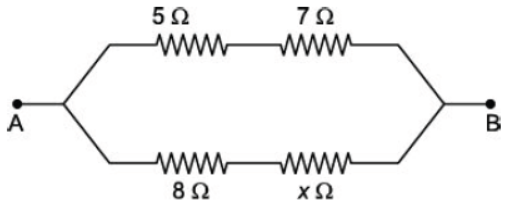Answer: The network of resistors can be simplified as (·.· 5 W and 7 W are in series and also 8 W and x W are in series).
Here, equivalent resistance (R) = 6 W, R1 = 12 W, R2 = (8 + x) W
The resistors R1 and R2 are connected in parallel.
∴ 1/R = 1/R1 + 1/R2
or 1/6 = 1/12 + 1/8 + x
or 1/6 - 1/12 = 1/8 + x
or 2 - 1/ 12 = 1/8 + x
or 1/12 = 1/8 + x
or 8 + x = 12
∴ x = 12 – 8 = 4 W.

Question. Calculate the equivalent resistance of the following network :
Answer: Let Rp is the equivalent resistance of resistors connected in parallel.
∴ Equivalent resistance of the circuit
1/Rp = 1/20 + 1/20
1/Rp = 1 + 1/20
= 2/20 = 1/10
Rp = 10 W
Now, equivalent circuit becomes.
˙.˙ 10 W and 10 W are connected in series.
∴ Equivalent resistance of the circuit R = 10 W + 10 W
= 20 W

Question. The circuit diagram given below shows the combination of three resistors, R1 = 2 W, R2 = 12 W and R3 = 6 W :
Calculate :
(a) Total resistance of the circuit.
(b) Total current flowing in the circuit.
(c) The potential difference across R1.
(d) The potential difference across R2 or R3.
(e) Current flowing through R2.
Answer: (a) R2 and R3 are in parallel.
∴ Equivalent resistance of R2 and R3
RP = R2 x R3/R2 + R3
= 12 x 6/ 12 + 6 Ω
= 72/18 Ω
= 4 W.
∴ Total resistance of the circuit, R = R1 + RP
Now, R1 and RP are connected in series
= (2 + 4) W
= 6 W
(b) Potential difference, V = 12 V
Total resistance, R = 6 W
Using Ohm’s law,
Total current flowing in circuit,
I = V/R
= 12/6 A = 2A
(c) Potential difference across R1,
V1 = IR1
= 2 × 2 = 4 V
(d) Potential difference across R2 or R3,
V2 = IRP
= 2 × 4 = 8 V
(e) Let the current flowing through R2 be I'.
∴ V2 = I'R2
or I' = V2/R2 = 8/12 A
= 0.67 A.

Question. In a network of resistors as shown in figure, calculate the equivalent resistance between the points (a) S and R and (b) P and R.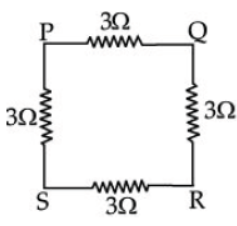Answer: (a) Between S and R : Between the points S and R the above network of resistors can be represented as :
Equivalent resistance of three 3 Ω resistors connected in series,
R1 = (3 + 3 + 3) = 9 Ω
Now, R1 and 3 Ω are connected in parallel.
∴ Equivalent resistance R can be calculated as
1/R = 1/9 + 1/3
= 1 + 3/9
= 4/9
or R = 4/9 Ω = 2.25 Ω
(b) Between P and R : Between the points P and R the network
of resistors can be represented as :
Equivalent resistance of two 3 Ω resistors connected in series is
(3 + 3) = 6 Ω
Now, two 6 Ω resistors are connected in parallel.
∴ Equivalent resistance is given by
1/R = 1/6 + 1/6
1/R = 1+ 1/6 = 2/6 = 1/3
= 3 W.

Question. A copper wire has diameter 0.5 mm and resistivity of 1.6 × 10–8 W m. What will be the length of this wire to make its resistance 10 W ? How much does the resistance change if the diameter is doubled ?
Answer: Here, diameter of wire = 0.5 mm, Resistivity (r ) = 1.6 × 10–8
W M, Resistance (R) = 1 W. Let the length of wire l and the
resistance when diameter is doubled be R1.
∴ Radius = 0.5mn/2 = 0.25 mm
= 0.25/1000 = 0.00025 m
We know that, R = p ,1/A
∴ l = RA/P = Rπr2/p
⇒ l = 10 x 3.14 x (0.00025)2/1.6 x 10 -8
l = 10 x 3.14 x 0.00025 x 0.00025/1.6 x 10 -8
⇒ l = 10 x 3.14 x 0.00000625 x 10 -8/1.6
l = 10 x 10-8 x 0.00000196250/1.6
= 196.26/1.6 = 122.65 m = 122.7 m
When diameter wire is doubled then,
Now diameter = 0.5 × 2 = 1 mm
∴ Radius = 1/2 mn = 0.5 mm
= 0.5 /1000m = 0.0005 m
∴ R1 = p , 1/A = P 1/πr2
= 1.6 ×10–8 × 122.7/3.14 x 0.0005 x 0.0005
= 1.6 ×10–8 x 122.7/3.14 x 0.000000 = 1.6 x 122.7/3.14 x 0.000000 x 10–8
= 196. 32/78.5 = 2.5 W

Question. Which uses more energy, a 250 W T.V. set in 1 hour or a 1200 W toaster in 10 minutes?
Answer: For T.V. Set : Power, P = 250 W
Time, t = 1 h
Electrical energy consumed = P × t
= 250 x 1/1000 kWh
= 0.25 kWh
For toaster : Power, P = 1200 W
Time, t = 10 minutes
= 10/60 h = 1/6 h
Electrical energy consumed = P × t
= 1200 x 1/1000 x 6 kWh
= 0.20 kWh
The T.V. set uses more energy (0.25 kWh) whereas the toaster uses less energy (0.20 kWh).

Question. When a 12 V battery is connected across an unknown resistor, there is a current of 2.5 mA in the circuit. Find the value of the resistance of the resistor.
Answer: Here, Potential difference, V = 12 V
Current, I = 2.5 mA = 2.5/1000 A = 0.0025 A
Using Ohm's law
Resistance, R = V/I = 12/0.0025 = 4800 W

Question : B1 B2 and B3 are three identical bulbs connected as shown in figure. When all the three bulbs glow, a current of 3A is recorded by the ammeter A.(i) What happens to the glow of the other two bulbs when the bulb B1 gets fused?
(ii) What happens to the reading of A1, A2, A3 and A when the bulb gets fused?
(iii) How much power is dissipated in the circuit when all the three bulbs glow together?
(i) Since B1, B2 and B3 are in parallel, the potential difference across each of them will remain same. So when the bulb B1 gets fused,B1 ,B2 and B3 have the same potential and continues with the same energy dissipated per second, i.e. they will glow continuously as they were glowing before.
(ii) Resistance of the parallel combination when all the three bulbs are glowingSince resistance of each arm is same and p.d. is also same, current divides them equally. So 1A current will pass through each bulb Bl and By
Therefore, ammeter A1 and A3 reads l A current while A2 will read zero and ammeter A read 2 A current.
(iii) In parallel, total power consumed
Peq=P1 +P2 +P3
So, when all the three bulbs glow together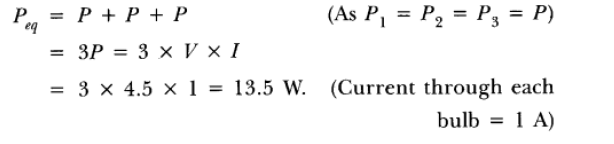Question : Find the current drawn from the battery by the network of four resistors Shown in the figure.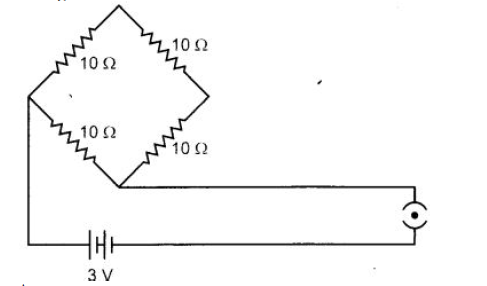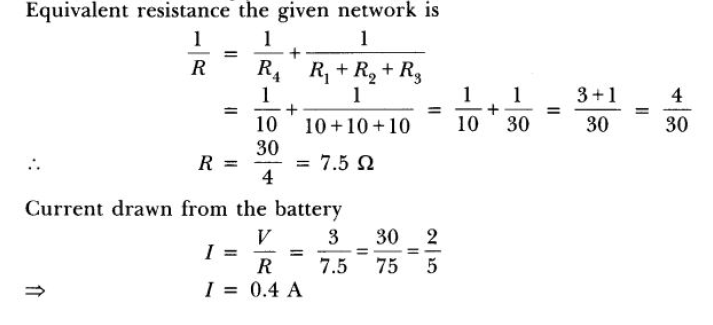Question : A 9Ω resistance is cut into three equal parts and connected in parallel. Find the equivalent resistance of the combination.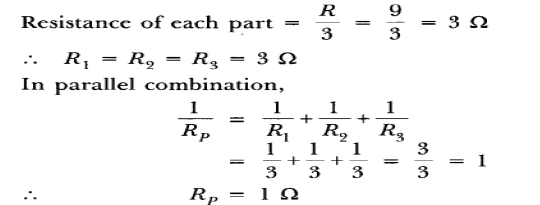Question : a.Two resistors Rand R2 may form (i) a series combination or (ii) a parallel combination, and the combination may be connected to a battery of 6 volts. In which combination, will the potential difference across R1 and across R2 be the same and in which combination, will the current through R1 and through R2 be the same?
b. For the circuit shown in this diagram, calculate(i) the resultant resistance.
(ii) the total current.
(iii) the voltage across 7 Ω resistor.

Answer. a. Potential difference across R1 and R2 is same in parallel combination of R1 and R2 and the current through R1 and R2 will be same when they are connected in series.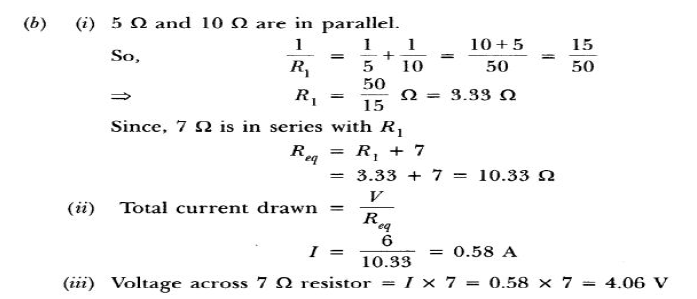CBSE Class 10 Science Chemical Reactions and Equations Assignment
 CBSE Class 10 Science Acids Bases and Salts Assignment Set A CBSE Class 10 Science Acids Bases and Salts Assignment Set B CBSE Class 10 Science Acids Bases and Salts Assignment Set C
 CBSE Class 10 Science Metals and Non Metals Assignment Set A CBSE Class 10 Science Metals and Non Metals Assignment Set B
 CBSE Class 10 Science Carbon and its compounds Assignment Set A CBSE Class 10 Science Carbon and its compounds Assignment Set B CBSE Class 10 Science Carbon and its compounds Assignment Set C CBSE Class 10 Science Carbon and its compounds Assignment Set D CBSE Class 10 Science Carbon and its compounds Assignment Set E
 CBSE Class 10 Science Periodic Classification Of Elements Assignment Set A CBSE Class 10 Science Periodic Classification Of Elements Assignment Set B CBSE Class 10 Science Periodic Classification Of Elements Assignment Set C
 CBSE Class 10 Science Biology Life Process Respiration Assignment CBSE Class 10 Science Biology Nutrition Assignment CBSE Class 10 Science Biology Respiration Assignment CBSE Class 10 Science Biology Transport Enrichment Assignment CBSE Class 10 Science Biology Transportation Assignment CBSE Class 10 Science Life Processes Assignment Set A CBSE Class 10 Science Life Processes Assignment Set B CBSE Class 10 Science Life Processes Assignment Set C
 CBSE Class 10 Science Control and Coordination Assignment Set A CBSE Class 10 Science Control and Coordination Assignment Set B CBSE Class 10 Science Control and Coordination Assignment Set C CBSE Class 10 Science Control and Coordination Assignment Set D
 CBSE Class 10 Science How do Organisms Reproduce Assignment Set A CBSE Class 10 Science How do Organisms Reproduce Assignment Set B
 CBSE Class 10 Science Heredity and Evolution Assignment Set A CBSE Class 10 Science Heredity and Evolution Assignment Set B CBSE Class 10 Science Heredity and Evolution Assignment Set C
 CBSE Class 10 Science Light Reflection and Refraction Assignment Set A CBSE Class 10 Science Light Reflection and Refraction Assignment Set B CBSE Class 10 Science Light Reflection and Refraction Assignment Set C CBSE Class 10 Science Light Reflection and Refraction Assignment Set D CBSE Class 10 Science Light Reflection and Refraction Assignment Set E CBSE Class 10 Science Light Reflection and Refraction Assignment Set F
 CBSE Class 10 Science Human Eye and Colorful World Assignment Set A CBSE Class 10 Science Human Eye and Colorful World Assignment Set B CBSE Class 10 Science Human Eye and Colorful World Assignment Set C
 CBSE Class 10 Physics Electricity Assignment Set A CBSE Class 10 Physics Electricity Assignment Set B CBSE Class 10 Physics Electricity Assignment Set C CBSE Class 10 Science Electricity Assignment Set A CBSE Class 10 Science Electricity Assignment Set B CBSE Class 10 Science Electricity Assignment Set C
 CBSE Class 10 Science Magnetic Effects of Electric Current Assignment Set A CBSE Class 10 Science Magnetic Effects of Electric Current Assignment Set B CBSE Class 10 Science Magnetic Effects of Electric Current Assignment Set C CBSE Class 10 Science Magnetic Effects of Electric Current Assignment Set D
 CBSE Class 10 Science Sources of Energy Assignment Set A CBSE Class 10 Science Sources of Energy Assignment Set B CBSE Class 10 Science Sources of Energy Assignment Set C CBSE Class 10 Science Sources of Energy Assignment Set D
 CBSE Class 10 Science Our Environment Assignment Set A CBSE Class 10 Science Our Environment Assignment Set B CBSE Class 10 Science Our Environment Assignment Set C
 CBSE Class 10 Science Sustainable Management of Natural Resources Assignment Set A CBSE Class 10 Science Sustainable Management of Natural Resources Assignment Set B CBSE Class 10 Science Sustainable Management of Natural Resources Assignment Set C
 CBSE Class 10 Physics Revision Assignment Set A CBSE Class 10 Physics Revision Assignment Set B CBSE Class 10 Physics Revision Assignment Set C CBSE Class 10 Physics Revision Assignment Set D CBSE Class 10 Physics Revision Assignment Set E CBSE Class 10 Physics Revision Assignment Set F CBSE Class 10 Physics Revision Assignment Set G CBSE Class 10 Physics Revision Assignment Set H
 CBSE Class 10 Science Assignment CBSE Class 10 Science Assignments Collection CBSE Class 10 Science Energy Crossword Puzzle Assignment
 CBSE Class 10 Physics Question Bank CBSE Class 10 Physics Tungsten Assignment
 CBSE Class 10 Science Chemistry Cleaning Capacity of Soap Assignment CBSE Class 10 Science Chemistry Determination of pH Assignment CBSE Class 10 Science Chemistry Different Types of Chemical Reactions Assignment CBSE Class 10 Science Chemistry Displacement Reaction and Reactivity Series Assignment CBSE Class 10 Science Chemistry Properties of Acetic Acid Assignment CBSE Class 10 Science Chemistry Saponification Preparation of Soap Assignment CBSE Class 10 Science Chemistry Study of properties of HCI Acid and NaOH Assignment

Tags